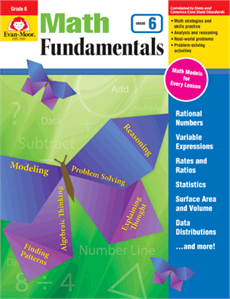# Math Fundamental - Grade 6

• \$26.99
Unit price per
Shipping calculated at checkout.

Comprehensive but not complicated! Math Fundamentals helps your sixth graders navigate the new math. Math Models and analysis questions, plenty of skill practice, and real-world problems guide students in thinking through and solving problems.

To help you target instruction, each unit clearly lists the standards information, mathematical practices, and skills covered.

Within a unit, math lessons are presented simply. Every math lesson includes:
• A Math Models reference page that shows students strategies for solving problems. This is helpful for parents and students transitioning to new math.
• Skill practice pages that progress in difficulty.
• A culminating problem-solving task that leads students through the process of solving a real-life problem.
• Use ratios to solve problems
• Divide with fractions
• Compute with decimals and find factors and multiples
• Use positive and negative numbers and absolute value
• Write and evaluate expressions
• Solve equations and inequalities
• Use dependent and independent variables
• Solve area, surface area, and volume problems
• Understand statistical measures
• Use data distributions

Designed to support new math programs, Math Fundamentals is the perfect easy-to-use supplement to your Common Core math or current math curriculum.
Better together! Use Math Fundamentals with Daily Math Practice for complementary skill practice that ensures your students master grade-level math.
This resource contains teacher support pages, reproducible student pages, and an answer key.